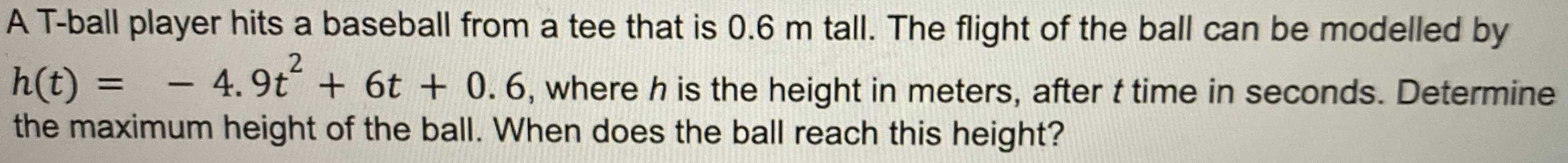### ¿Todavía tienes preguntas de matemáticas?

Pregunte a nuestros tutores expertos
Algebra
PreguntaA T-ball player hits a baseball from a tee that is $$0.6 m$$ tall. The flight of the ball can be modelled by

$$h ( t ) = - 4.9 t ^ { 2 } + 6 t + 0.6 ,$$ where $$h$$ is the height in meters, after $$t$$ time in seconds. Determine the maximum height of the ball. When does the ball reach this height?